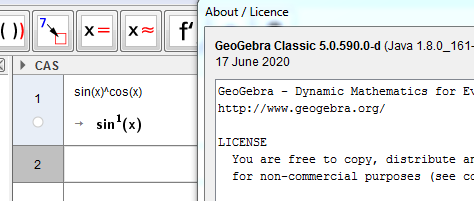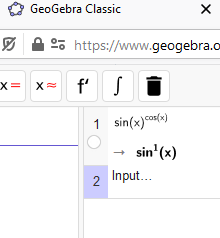# Geogebra gives wrong derivative for following function f(x)=sin(x)^cos(x

kaisa123 shared this question 2 years ago

Geogebra gives wrong derivative for following simple function f(x)=sin(x)^cos(x). It gives f'(x)=cos(x)(I have Lenovo and windows 10). My sister's (macbook) gives the right derivative f'(x)=-ln(sin(x))*sin^(cos(x)+1)+cos(x)^2*sin(x)^(cos(x)-1).1

GG Classic 5 and 6 both give the right derivate. The Graphic Calculator produces the right graph for it. So what do you mean with "Geogebra produces"? At least we know your sister is using a macbook and you're using windows 10, but which version or app of GeoGebra are you using? This is what GG 5 & 6 Classic give me on a my laptop with win10.1

I confirm that

ggb6n.jpg - before pressing Enter

ggb6na.jpg - after pressing Enter1

First, I just said I got the right derivate and asked about what version kaisa was using, since there was just a description and no file nor screen capture picture.

Second I us the same GG5 as you, but the position of cos in the equation is different.

For the different result in GG6 I've got no explanation.1

In the CAS window1

and in Geogebra 6 too1

efectivamente el CAS transforma cualquier expresion que resulte sin(x)^cos(x) en sin(x)^1 incluso la expresion sin(x)^(cotan(x)*sin(x)) es transformada

creo recordar que este bug ya estuvo entre nosotros hace seis meses y parece que ahora vuelve1

This is definitely a bug. It is at least in GG5, GG6, GeoGebra CAS and Calcultor Suite.

M11

problem persist in ver5also online1

Sorry, my mistake. Should be in v5911

v591 online now1

Thanks, works OK in GeoGebra 5.